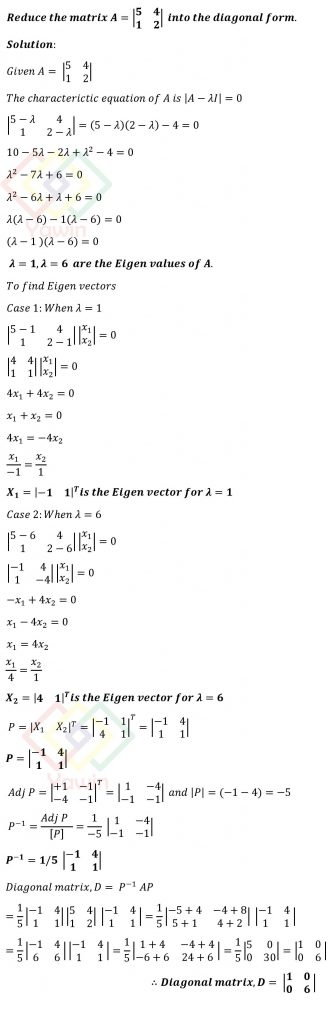Reduce the matrix A=[(5,4),(1,2)] into the diagonal form

## Question## Question

Reduce the matrix A=[(5,4),(1,2)] into the diagonal form

## Topic

Diagonalisation of a Square Matrix